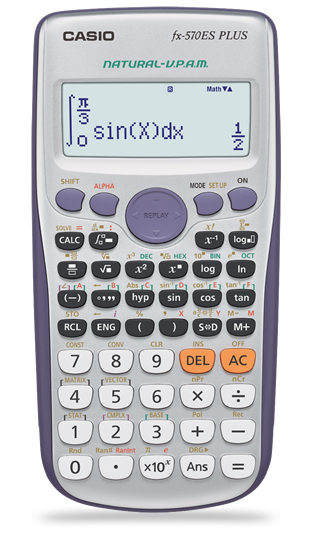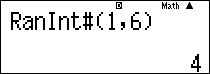Home > Products > Natural Textbook Diplay Models > fx-570ES PLUS

# fx-570ES PLUS

Overview# Software features

• ### New equation mode(√and fraction display)Click to watch animation
• ### Random integersClick to watch animation

## Other features

• One AAA-size(R03) battery
• 417 Functions
• Full Dot Display
• Plastic Keys
• Integration / Differential
• Equation calculation
• Matrix calculations / Vector calculations
• Complex number calculation
• CALC function / SOLVE function
• Base-n calculation
• Fraction calculation
• Combination and Permutation
• Logarithm log
• List-based STAT data editor
• Standard deviation
• Paired-variable statistics regression analysis
• Logical operations
• Table function
• 40 scientific constants
• 40 metric conversions (20 conversion pairs)
• 9 variable memories
• Comes with new slide on hard case